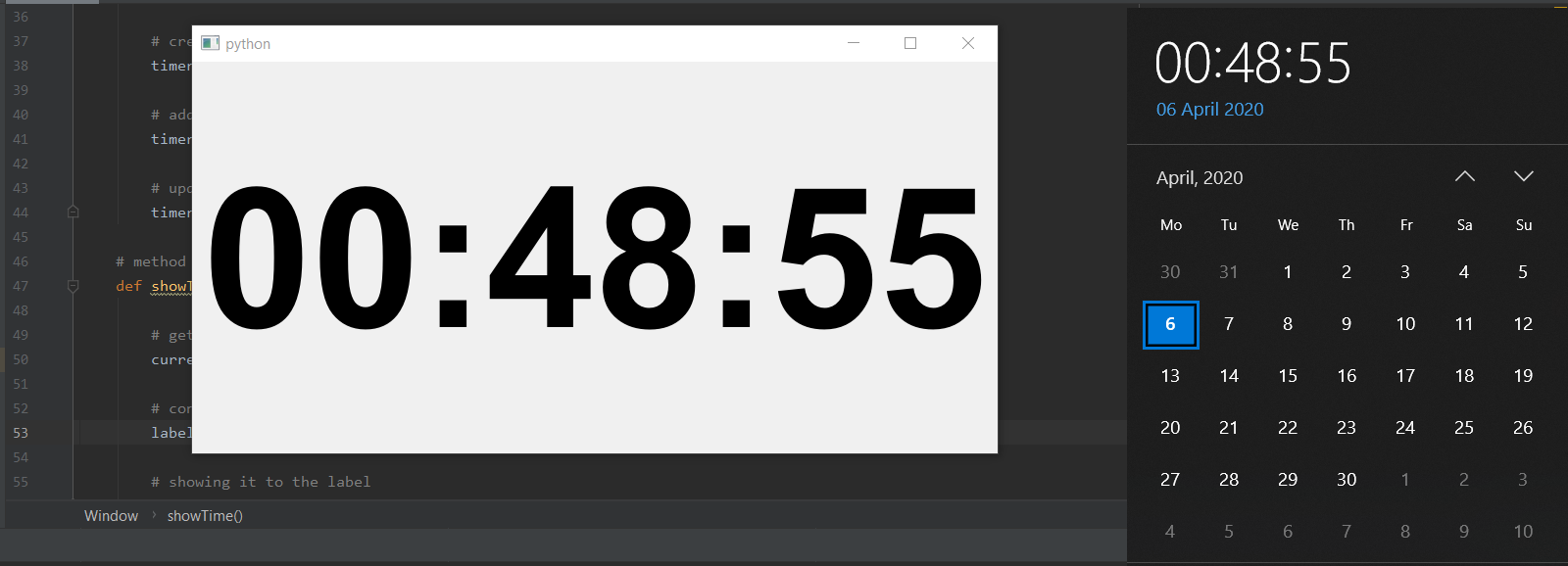# PyQt5 – Create a digital clock

• Last Updated : 22 Apr, 2020

In this article we will see how to create a digital clock using PyQt5, this digital clock will basically tells the current time in the 24 hour format.

In order to create a digital clock we have to do the following:

Attention geek! Strengthen your foundations with the Python Programming Foundation Course and learn the basics.

To begin with, your interview preparations Enhance your Data Structures concepts with the Python DS Course. And to begin with your Machine Learning Journey, join the Machine Learning - Basic Level Course

1. Create a vertical layout
2. Create label to show the current time and put it in the layout and align it to the center.
3. Create a QTimer object
4. Add action to the QTimer object such that after every 1sec action method get called.
5. Inside the action method get the current time and show that time with the help of label.

Below is the implementation –

 `# importing required librarie``import` `sys``from` `PyQt5.QtWidgets ``import` `QApplication, QWidget``from` `PyQt5.QtWidgets ``import` `QVBoxLayout, QLabel``from` `PyQt5.QtGui ``import` `QFont``from` `PyQt5.QtCore ``import` `QTimer, QTime, Qt`` ` ` ` `class` `Window(QWidget):`` ` `    ``def` `__init__(``self``):``        ``super``().__init__()`` ` `        ``# setting geometry of main window``        ``self``.setGeometry(``100``, ``100``, ``800``, ``400``)`` ` `        ``# creating a vertical layout``        ``layout ``=` `QVBoxLayout()`` ` `        ``# creating font object``        ``font ``=` `QFont(``'Arial'``, ``120``, QFont.Bold)`` ` `        ``# creating a label object``        ``self``.label ``=` `QLabel()`` ` `        ``# setting centre alignment to the label``        ``self``.label.setAlignment(Qt.AlignCenter)`` ` `        ``# setting font to the label``        ``self``.label.setFont(font)`` ` `        ``# adding label to the layout``        ``layout.addWidget(``self``.label)`` ` `        ``# setting the layout to main window``        ``self``.setLayout(layout)`` ` `        ``# creating a timer object``        ``timer ``=` `QTimer(``self``)`` ` `        ``# adding action to timer``        ``timer.timeout.connect(``self``.showTime)`` ` `        ``# update the timer every second``        ``timer.start(``1000``)`` ` `    ``# method called by timer``    ``def` `showTime(``self``):`` ` `        ``# getting current time``        ``current_time ``=` `QTime.currentTime()`` ` `        ``# converting QTime object to string``        ``label_time ``=` `current_time.toString(``'hh:mm:ss'``)`` ` `        ``# showing it to the label``        ``self``.label.setText(label_time)`` ` ` ` `# create pyqt5 app``App ``=` `QApplication(sys.argv)`` ` `# create the instance of our Window``window ``=` `Window()`` ` `# showing all the widgets``window.show()`` ` `# start the app``App.exit(App.exec_())`

Output :My Personal Notes arrow_drop_up